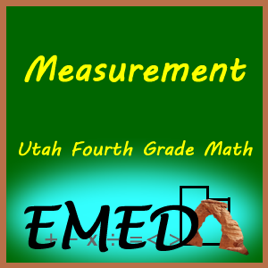48 Folgen

This is a feed of pages for Measurement# Measurement Utah Electronic High School

• Kurse

This is a feed of pages for Measurement

• video
4.2f Perimeter

## 4.2f Perimeter

f. Determine possible perimeters, in whole units, for a rectangle with a fixed area, and determine possible areas when given a rectangle with a fixed perimeter.

• video
4.1a Relative size of metric units – length, capacity, weight

## 4.1a Relative size of metric units – length, capacity, weight

a. Describe the relative size among metric units of length (i.e., millimeter, centimeter, meter), between metric units of capacity (i.e., milliliter, liter), and between metric units of weight (i.e., gram, kilogram).

• video
4.1c Estimate/measure capacity

## 4.1c Estimate/measure capacity

c. Estimate and measure capacity using milliliters, liters, cups, pints, quarts, and gallons, and measure weight using grams and kilograms.

• video
4.1b Relative size of customary units - capacity

## 4.1b Relative size of customary units - capacity

a. Describe the relative size among customary units of capacity (i.e., cup, pint, quart, gallon).

• video
4.2e Develop relationships among area formulas

## 4.2e Develop relationships among area formulas

Develop/use relationships among area formulas of triangles and parallelograms to right triangles/rectangles

• video
4.1d Develop benchmark angles – 45°, 60°, 120° – using 90° angles

## 4.1d Develop benchmark angles – 45°, 60°, 120° – using 90° angles

d. Recognize that angles are measured in degrees and develop benchmark angles (e.g., 45°, 60°, 120°) using 90° angles to estimate angle measurement.